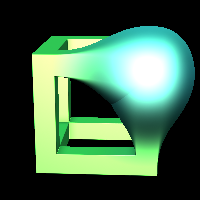# combine

Shortcuts:
• cmb
Keywords:
• combine
• diff
• intersect
• union
combine
combine
RayTK
v0.36

Combines SDFs in various ways.

Depending on which `Combine` option is selected, different parameters will be enabled. This operator only supports two input SDFs (along with a value field to control blending). To combine more than two SDFs, use one of the specialized operators like `simpleUnion`.

## Parameters

• `Enable`:
• `Combine`: The type of combination operation to perform.
• `Simple Union`: The combined areas of each of the inputs.
• `Simple Intersect`: The overlapping areas of each of the inputs.
• `Simple Difference`: The first input with the second input removed from it.
• `Smooth Union`: Like `simpleUnion` but with the intersecting edges rounded out.
• `Smooth Intersect`: Like `simpleIntersect` but with the intersecting edges rounded out.
• `Smooth Difference`: Like `simpleDiff` but with the intersecting edges rounded out.
• `Round Union`: Uses a quarter circle blending area along the edges.
• `Round Intersect`:
• `Round Difference`:
• `Chamfer Union`: Uses a 45 degree flat slope to blend along the edges.
• `Chamfer Intersect`:
• `Chamfer Difference`:
• `Stair Union`: Uses vertical and horizontal stairs to blend along the edges.
• `Stair Intersect`:
• `Stair Difference`:
• `Column Union`: Uses multiple circular tubes to blend along the edges.
• `Column Intersect`:
• `Column Difference`:
• `Swap Inputs`: Swaps the order of the inputs. This is only relevant for "diff" modes.
• `Radius`: The size of the blending region.
• `Number`: For stair and column modes, this controls how many steps are used in the blending regions.
• `Offset`:

## Inputs

• `SDF 1`: (required)
• Coordinate Types: `float` `vec2` `vec3` `vec4`
• Context Types: `Context` `MaterialContext` `CameraContext` `LightContext` `RayContext` `ParticleContext`
• Return Types: `Sdf`
• `SDF 2`: (optional)
• Coordinate Types: `float` `vec2` `vec3` `vec4`
• Context Types: `Context` `MaterialContext` `CameraContext` `LightContext` `RayContext` `ParticleContext`
• Return Types: `Sdf`
• `Radius Field`: (optional)
• Coordinate Types: `float` `vec2` `vec3` `vec4`
• Context Types: `Context` `MaterialContext` `CameraContext` `LightContext` `RayContext` `ParticleContext`
• Return Types: `float` `Sdf`
• `Offset Field`: (optional)
• Coordinate Types: `float` `vec2` `vec3` `vec4`
• Context Types: `Context` `MaterialContext` `CameraContext` `LightContext` `RayContext` `ParticleContext`
• Return Types: `float`